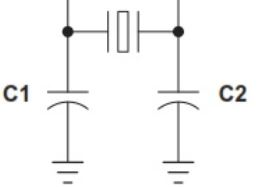# How to calculate the actual load capacitance for crystal on a MCU.Crystal is on the most often used clock source for micro-controller. It requires 2 capacitor with same value(typically) connect between the 2 terminals of the crystal and the ground  in order to match the load capacitance of the crystal that you are using.

Here is the how you calculate the values of load capacitors used for crystal:

Load Capacitance = [(Cd x Cg)/(Cd+Cg)]+Cic+△C

where Cd and Cg are the 2 capacitor you placed on each side of the crystal. Cic is the capacitance of IC, usually 3-5pF experimentally. and I usually ignore △C as the capacitance on PCB since no high speed device is involved.

Since Cd ss same as Cg most likely, we can simplify the equation to:

Load Capacitance = [(Cd x Cd)/(Cd+Cd)]+Cic = Cd/2+Cic

therefore Cd = (LC – Cic) x 2

If Lc is 8pF, I say Cic is 3-5pF, Cd will be 6-10pF

most of the CL for crystal running at 8MHz(normally used for stm32) I ever used are 18pf, in this case, the Cd will be 26-30pF. However, it can run with lower capacitance depends on the quality of crystal since I made it worked at 18pF capacitors before. If you don’t have the exact value of capacitor needed for crystal, higher is better than lower value just in case it’s too low to make the crystal oscillate.